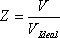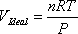# 7.1: Z-Factor

From the last module, it is very likely that one question is left in our minds. How can we adjust the ideal model to make it suitable for real gases? Well, we already have the answer. We said that once we have established a base (ideal) model, we look at a real case by estimating how close (or far) it performs with respect to the base (ideal) case, and introducing the corresponding corrections. Again, such corrections will take into account all the considerations that our original assumptions left out.

For the case of gas behavior, we introduce a correction factor to account for the discrepancies between experimental observations and predictions from our ideal model. This correction factor is usually referred to as the compressibility factor(Z), and is defined as:(7.1)

In the previous equation, V is the real volume occupied by the gas and VIdeal is the volume that the ideal model predicts for the same conditions. The ideal volume is given by:(7.2)

Hence, the equation of state for real gases is written as:(7.3)

Engineers are very much familiar with this equation, to the extent that it is usually recognized as Engineering EOS. Please note that for Z = 1, this equation collapses to the ideal gas model. In fact, unity is the compressibility factor of any gas that behaves ideally. However, please note that Z = 1 is a consequence of ideal behavior, but this is not a definition.